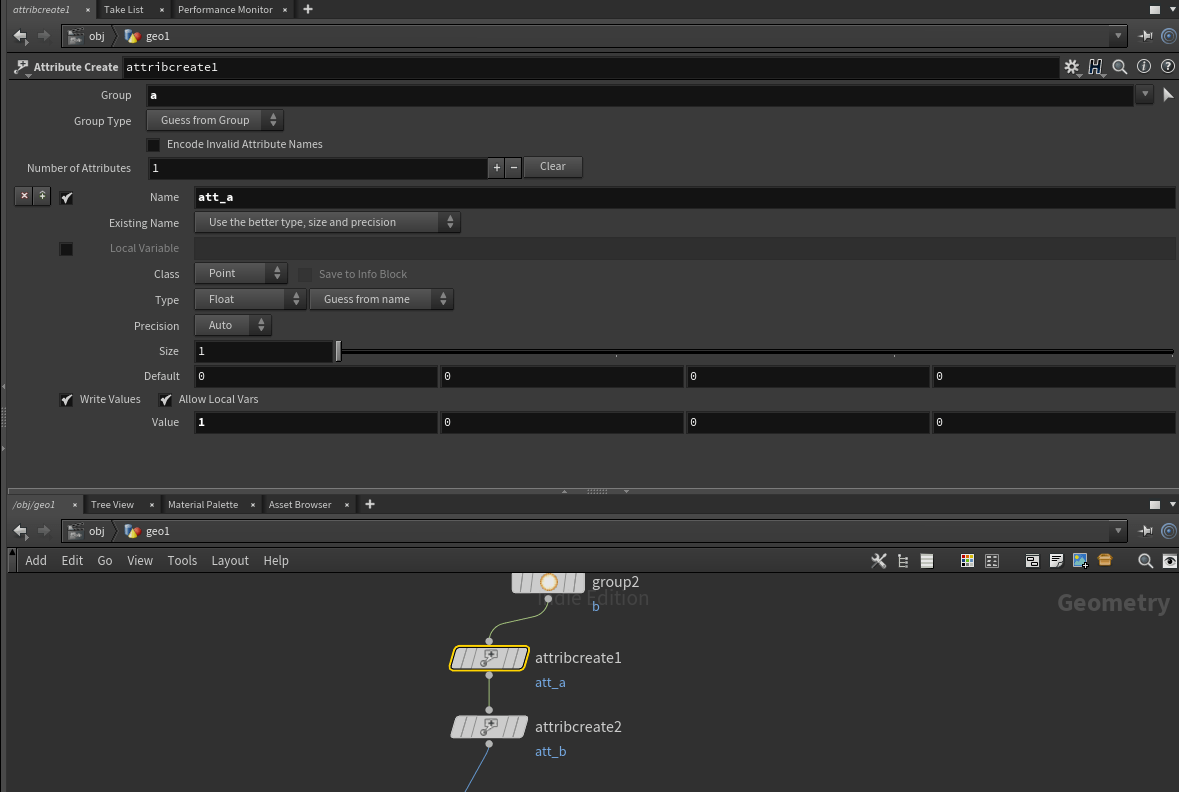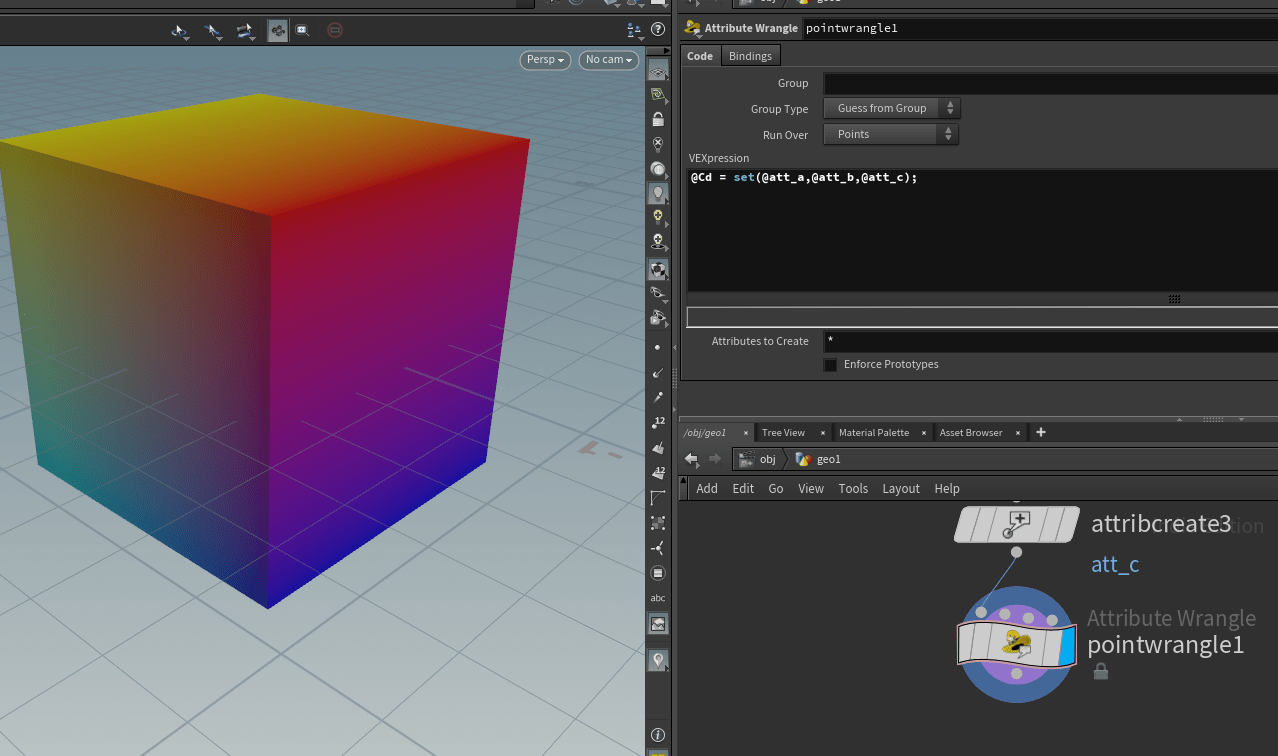## Houdini – Set point color by reading custom point attributes

Software:
Houdini 18.0.499

Took me some time to figure out how to set the points color (“Cd”) attribute with data stored initially in custom points attributes.
I kept trying to use the Color SOP node with a “point()” function in its R, G, B fields attempting to refer to the wanted attributes but it didn’t work for me,
I also tried various loop setups iterating the geometry points, couldn’t get that to work either..
* I’m new to Houdini so the fact these approaches didn’t work for me doesn’t mean they can’t be used for this..

I finally managed to do this using a Point Wrangle node with the following VEX expression that sets the Cd (color) attribute’s vector components by referring to the 3 custom attributes “att_a”, “att_b” and “att_c” (see image below):

`@Cd = set(@att_a,@att_b,@att_c);`

What the Point Wrangler node does that I couldn’t achieve by writing expressions into the RGB fields of the Color node or by using loops is that it iterates all its input SOP’s points, and within its expression the attribute name i.e. “att_a” etc. automatically refers to that named attribute in the same point that is now being iterated over.

Note:
The reason I need such a workflow in the first place is to generate geometric property masks for a Houdini asset, that will be available for the target shading system via vertex color input.
* The Houdini point color attribute propagates to vertex color on output.A custom “att_a” point attribute is added to a group of points using the Attribute Create SOP nodeThe Point Wrangler node with its expression

After setting the point color, I added an Attribute Delete SOP node to delete the no more necessary custom attributes:

## Blender – Vertex Paint Selected Vertices

Software:
Blender 2.82

1. In Edit Mode, Select the wanted vertices.
2. In Vertex Paint Mode,
Activate the Vertex Selection option:3. Use the brush to paint or Shift + K to set the selected vertices color.

## Python for 3ds max – Mesh manipulation

Software:
3ds max 2019

An example of creating a mesh ripple deformation using Python for 3ds max:Script steps:

1. Define the effect intensity and a helper point object that will serve as the effect center.
2. Collapse the object to an Editable-Mesh so its vertices will be accessible by Python.
Note that the Node‘s Object has to be cast as a TriObject, to access the object’s Mesh data.
3.  Loop through the Mesh’s vertices, get their world position, and set a new Z position as a sine function of the distance from the effect center.
```import math
from MaxPlus import Factory
from MaxPlus import ClassIds
from MaxPlus import INode
from MaxPlus import TriObject
from MaxPlus import Matrix3
from MaxPlus import Point3

# Intensity:
effecr_mult = 1.0

# Effect center:
effector = INode.GetINodeByName('Point001')
effect_pos = effector.GetWorldPosition()

# Prepare object and eccess it's mesh data:
node = INode.GetINodeByName('Teapot001')
new_edit_mesh_mod = Factory.CreateObjectModifier(ClassIds.Edit_Mesh)
node.Collapse(True)
node_tm = node.GetWorldTM()
node_pos = node.GetWorldPosition()
obj = node.GetObject()
triobj = TriObject._CastFrom(obj)
mesh = triobj.GetMesh()

# Process the object's vertices:
for i in range(mesh.GetNumVertices()):
# Get vertex in world space
vert_pos = mesh.GetVertex(i)
vert_world_pos = node_tm.VectorTransform(vert_pos)
vert_world_pos = vert_world_pos + node_pos
# Get vertex distance from effect center:
diff_vec = vert_world_pos - effect_pos
diff_vec.Z = 0
dist = diff_vec.GetLength()
# Set new vertex position:
mesh.SetVert(i,vert_pos.X,vert_pos.Y,vert_pos.Z + math.sin(dist)*effecr_mult)```

* note that when copying and pasting a script from this example, the indentation may not be pasted correctly.

Related Post:
Python for 3ds max – Animated Mesh

## Python for 3ds max – Animated Mesh

Software:
3ds max 2019

This is an example of procedurally animating a mesh’s vertices via Python script.Notes:
1. The model has to be converted to Editable Mesh before the script is run.
* unless the scrip will be extended to do it.
2. The model must be selected for the script to work.

```import MaxPlus
import math
from MaxPlus import INode
from MaxPlus import TriObject
from MaxPlus import SelectionManager
from MaxPlus import Factory
from MaxPlus import Animation
from MaxPlus import Point3
from MaxPlus import Control

ticks_frame = 160

#Selection
sel = []
for n in SelectionManager.Nodes:
sel.append(n)
node = sel

#Setup Controllers
obj = node.GetObject()
Tri = TriObject._CastFrom(obj)
mesh = Tri.GetMesh()
num_verts = mesh.GetNumVertices()
mesh_anim = obj.GetSubAnim(0)
pnt_ctrl = Factory.CreateDefaultMasterPointController()
node.AssignController(pnt_ctrl,1)
for i in range(num_verts):
bezp3 = Factory.CreateDefaultPoint3Controller()
bezp3.SetPoint3Value(mesh.GetVertex(i))
mesh_anim.AssignController(bezp3,i)

#Animation
Animation.SetAnimateButtonState(True)
for t in range(100):
time = t * ticks_frame
Animation.SetTime(time)